PBR学习个人总结(一)

# 一、 PBR知识体系架构图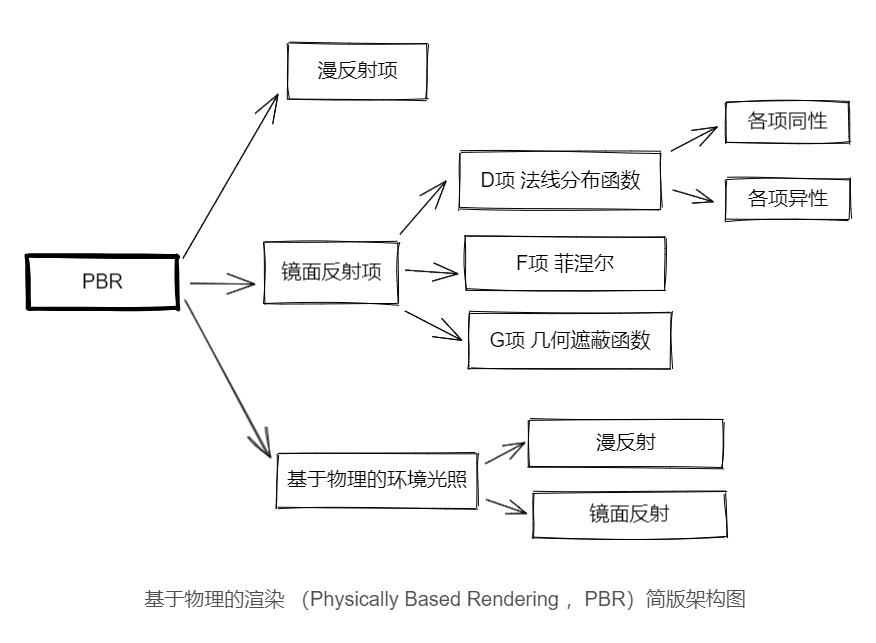清晰了上图的架构之后 ，就可以按各分支详细分解了。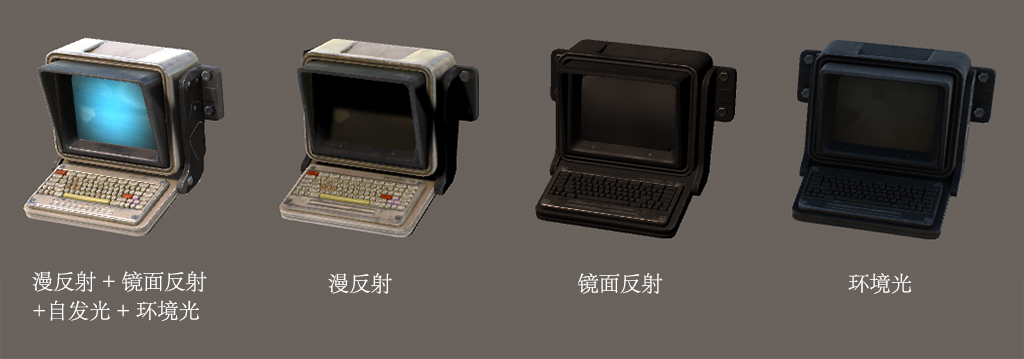# 二、资源列表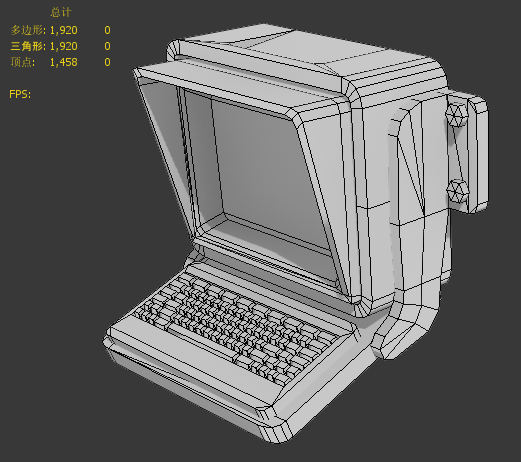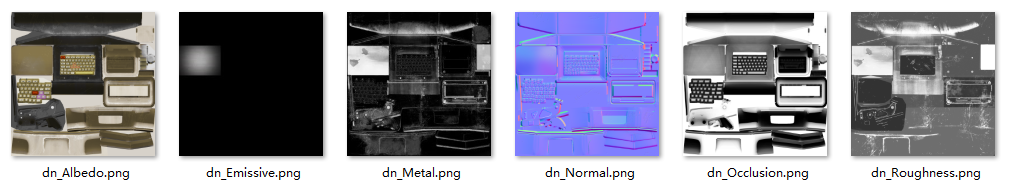Properties属性内容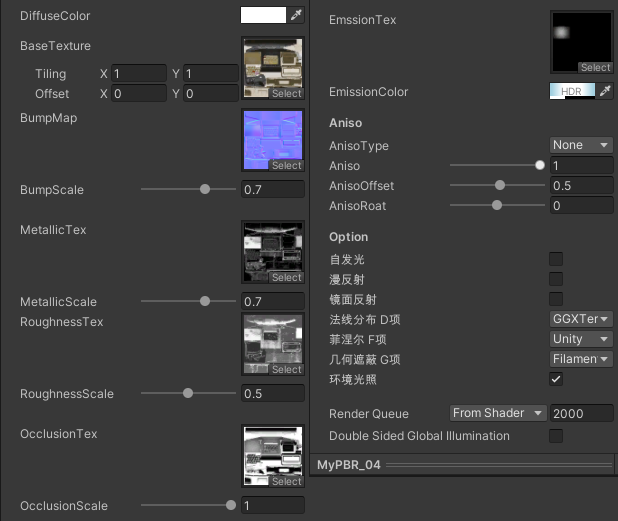```Properties
{
_DiffuseColor("DiffuseColor",Color)=(1,1,1,1)
[Space(10)]_MainTex ("BaseTexture", 2D) = "white" {}
[NoScaleOffset]_BumpMap("BumpMap",2D)="white"{}
_BumpScale("BumpScale",Range(0,1))=1

[Space(20)]
[NoScaleOffset]_MetallicTex("MetallicTex",2D)="white"{}
_MetallicScale("MetallicScale",Range(0.0,1.0))=0.0
[NoScaleOffset]_RoughnessTex("RoughnessTex",2D)="white"{}
_RoughnessScale("RoughnessScale",Range(0,1))=0.5

[Space(20)] [NoScaleOffset]_OcclusionTex("OcclusionTex",2D)="white"{}
_OcclusionScale("OcclusionScale",Range(0,1))=1

[Space(10)] [NoScaleOffset]_EmssionTex("EmssionTex",2D)="white"{}
[HDR]_EmissionColor("EmissionColor",Color)=(1,1,1)

[Space(10)]
[KeywordEnum(None,GTR2,GGX)] _ANISO("AnisoType",float)=0

_Aniso("Aniso",Range(0,1))=0
_AnisoOffset("AnisoOffset",Range(-10,10))=0
_AnisoRoat("AnisoRoat",Range(-10,10))=0
// [Space(10)] _ClearCoat("ClearCoat",Range(0,1))=0
[Space(10)]
[Toggle] _IS_AMBIENT("自发光",float)=1
[Toggle] _IS_DIFFUSE("漫反射",float)=1
[Toggle] _IsSpecular("镜面反射",float)=1
[KeywordEnum(None,GGXTerm,GTR1,GTR2,GND)] _UIDNF("法线分布 D项",float)=0
[KeywordEnum(None,Unity,Schlick,SG)] _UIF("菲涅尔 F项",float)=0
[KeywordEnum(None,Filament,GGX,GSF,Beckman)] _UIG("几何遮蔽 G项",float)=0
[Toggle] _ISIBL("环境光照",float)=1
}```

# 三、漫反射项

## 3.1 漫反射和镜面反射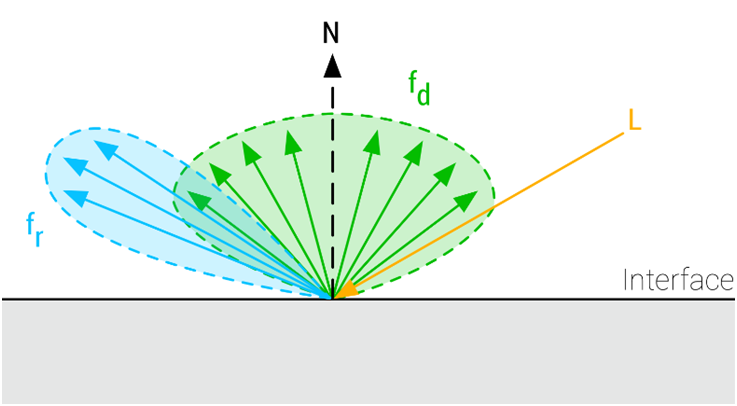## 3.2公式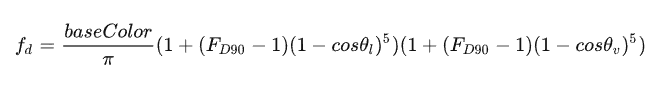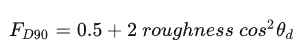## 3.3效果展示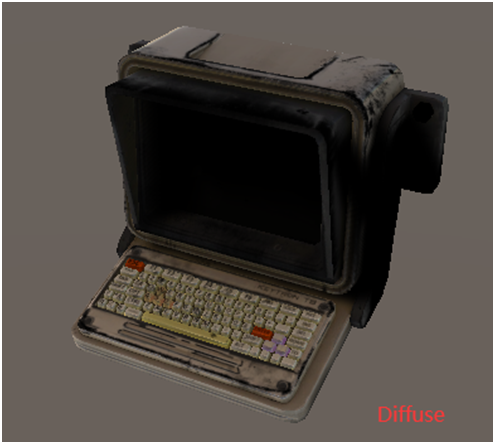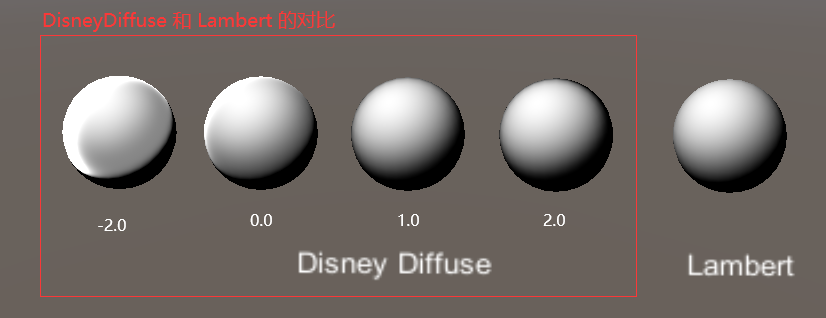左侧为是显示明显的对比，把阀值范围设成-2~2之间

## 3.4 相关代码

```half DisneyDiffuse(half NdotV, half NdotL, half LdotH, half perceptualRoughness)
{
half fd90 = 0.5 + 2 * LdotH * LdotH * perceptualRoughness;
// Two schlick fresnel term
half lightScatter   = (1 + (fd90 - 1) * Pow5(1 - NdotL));
half viewScatter    = (1 + (fd90 - 1) * Pow5(1 - NdotV));
return lightScatter * viewScatter;
}
inline half Pow5 (half x){
return x*x * x*x * x;
}```

Pow5这个方法，有一维到四维，但为什么不直接用pow函数，而是使用相同的量相乘5次，其在文中也有说到其两个好处：

1.   更好的优化渲染管线

2.   不用担心出现异常

【基于物理的渲染（PBR）白皮书】系列